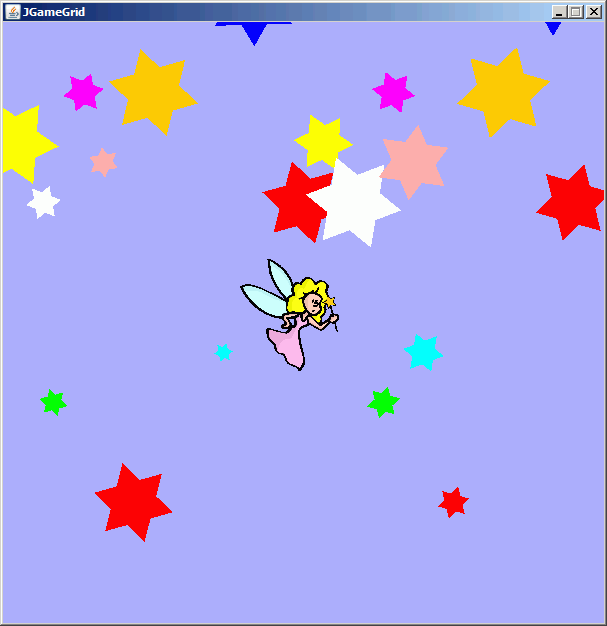JGameGridThe source code of all examples is included in the JGameGrid distribution.

Ex11: Background Painting

In JGameGrid you have full access to the background offscreen buffer where you can draw figures using the well-known Java Graphics2D library. In every simulation cycle this buffer is rendered to the screen before all actor images, by default the background is just an empty black surface. The background painting is made easy by the GGBackground class where you find many of the drawing methods of Graphics2D in a simplified version. The size of the background is determined by the number of horizontal cells (i), the number of vertical cells (k) and the cell size (s) of the GameGrid resp. GGPane window. Because 1 pixel is added to the right and the buttom to show the border cells completely, the size of the background in number of pixels is i * s + 1 horizontally and k * s + 1 vertically. For the background, pixel coordinates are used ranging from 0 to i * s (inclusive) horizonally and from 0 to k * s (inclusive) vertically.

E.g. constructing new GGPane(300, 200, 2) will give a background with 601 x 401 pixels. x-pixel coordinates are in the range 0 <= x <= 600 and y-pixel coordinates in the range 0 <= y <= 400.

Because GGBackground has no public constructor you must request the background reference by getBg() from the GameGrid or GGPane classes.

In the following example we compose a paradise with rotating and moving stars and an angel passing by. To draw the stars using Graphics2D in real-time gives you more flexibility than using actor images but requires more mathematical and programming skills. A star consists of two equilateral triangles rotated by 180 degrees. We use a general path for the triangle and an affine transformation to rotate it. The method turn() rotates the star by a given angle and showAt() draws the star into the background using the fillGeneralPath() methods.

 import java.awt.geom.*; import java.awt.*; import ch.aplu.jgamegrid.*; public class Star {   private GGBackground bg;   private Color color;   private GeneralPath tri1, tri2; // 2 triangles at (0,0)   public Star(GGBackground bg, int radius, Color color)   {     this.bg = bg;     this.color = color;     setSize(radius);   }   private void setSize(int size)   {     // First triangle, size is radius of circumcircle, center at (0,0)     tri1 = new GeneralPath();     tri1.moveTo(size, 0);     tri1.lineTo((int)(-0.5 * size), (int)(0.866 * size));     tri1.lineTo((int)(-0.5 * size), (int)(-0.866 * size));     tri1.closePath();     // Second triangle like first, but rotated 180 degrees     tri2 = (GeneralPath)tri1.clone();     AffineTransform t = new AffineTransform();     t.rotate(Math.PI);     tri2.transform(t);   }   public void turn(double angle)   {     AffineTransform t = new AffineTransform();     t.rotate(angle);     tri1.transform(t);     tri2.transform(t);   }   public void showAt(int mx, int my)   {     bg.setPaintColor(color);     AffineTransform t = new AffineTransform();     t.translate(mx, -50 + my % 650);  // Restrict to playground     // Cloning to avoid side effects     GeneralPath gp1 = (GeneralPath)tri1.clone();     GeneralPath gp2 = (GeneralPath)tri2.clone();     gp1.transform(t);     gp2.transform(t);     bg.fillGeneralPath(gp1);     bg.fillGeneralPath(gp2);   } }

The angel is an actor the moves over the window and is mirrored at the window borders.

 import ch.aplu.jgamegrid.*; public class Angel extends Actor {   public Angel()   {     super("sprites/angel.gif");   }   public void act()   {     Location loc = getLocation();     double dir = getDirection();     if (loc.x < 50 || loc.x > 550)     {       setDirection(180 - dir);       if (loc.x < 50)       {         setX(55);         setHorzMirror(false);       }       else       {         setX(545);         setHorzMirror(true);       }     }     if (loc.y < 50 || loc.y > 550)     {       setDirection(360 - dir);       if (loc.y < 50)         setY(55);       else         setY(545);     }     move();   } }

In the application class we create 20 different stars at different positions. Because the Angel class is responsible to move the angel we only need to animate the stars in the act() method of the application class. This is another demonstration how JGameGrid decouples different parts of an animated scenario.

 import ch.aplu.jgamegrid.*; import java.awt.Color; public class Ex11 extends GameGrid {   private final int nbStars = 20;   private Star[] stars = new Star[nbStars];   private final int mx[] =   {     10, 40, 50, 80, 100, 130, 150, 220, 250, 300,     320, 350, 380, 390, 410, 450, 500, 420, 550, 570   };   private final int my[] =   {     -10, 50, 250, -60, 10, 350, -60, 200, 500, 50,     -10, 50, 250, -60, 10, 350, -60, 200, 500, 50   };   private final double rotInc[] =   {     0.01, -0.02, 0.03, -0.02, 0.05, -0.1, 0.03, -0.02, -0.2, -0.1,     0.01, 0.02, -0.03, 0.02, -0.05, 0.1, -0.03, 0.02, 0.2, 0.1   };   private final Color colors[] =   {     Color.yellow, Color.white, Color.green,  Color.magenta, Color.pink,     Color.red, Color.orange, Color.cyan, Color.blue, Color.red,     Color.yellow, Color.white, Color.green,  Color.magenta, Color.pink,     Color.red, Color.orange, Color.cyan, Color.blue, Color.red   };   private GGBackground bg = getBg();   private int dy = 0;   public Ex11()   {     super(600, 600, 1, false);     setSimulationPeriod(50);     initStars();     addActor(new Angel(), new Location(300, 300), -22);     show();     doRun();   }   private void initStars()   {     int size;     for (int i = 0; i < nbStars; i++)     {       size = (int)(10 + 40 * Math.random());       stars[i] = new Star(bg, size, colors[i]);     }   }   public void act()   {     drawParadies();   }   private void drawParadies()   {     bg.clear(new Color(175, 175, 255));     for (int i = 0; i < nbStars; i++)     {       stars[i].turn(rotInc[i]);       stars[i].showAt(mx[i], my[i] + dy);     }     dy += 2;   }   public static void main(String[] args)   {     new Ex11();   } }

Execute the program locally using WebStart.

.